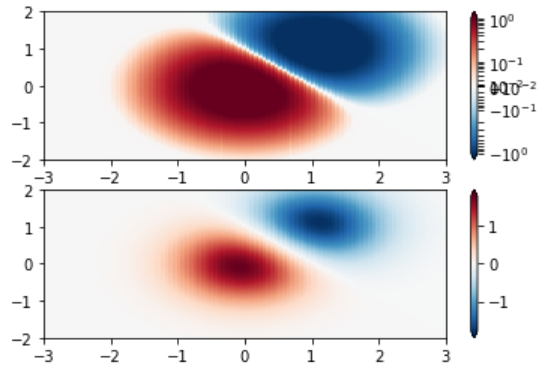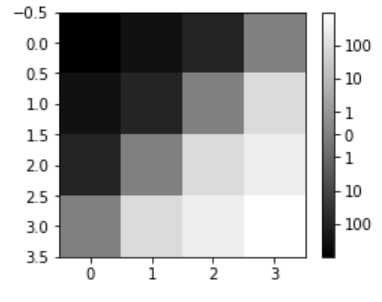# Matplotlib.colors.SymLogNorm class in Python

• Last Updated : 27 Jan, 2022

Matplotlib is an amazing visualization library in Python for 2D plots of arrays. Matplotlib is a multi-platform data visualization library built on NumPy arrays and designed to work with the broader SciPy stack.

Note: For more information, refer to Python Matplotlib – An Overview

## matplotlib.colors.SysLogNorm

The matplotlib.colors.SysLogNorm class belongs to the matplotlib.colors module. The matplotlib.colors module is used for converting color or numbers arguments to RGBA or RGB.This module is used for mapping numbers to colors or color specification conversion in a 1-D array of colors also known as colormap.

The matplotlib.colors.SymLogNorm class is used for symmetric logarithmic scaling to both positive and negative direction from the origin. As value close to the range of zero tend towards infinity, there rose a need to have a linear range around zero. The range under which the plot is linear is called linthresh. The linear range (-linthresh to +linthresh) is stretched relative to the logarithmic range by the help of linscale. The number of decades to use for each half of the linear range is its value. For instance if linscale==1.0(this is also the default), the space covered for the positive and negative halves of the linear range is equivalent to one decade in the range that is logarithmic in nature.

Methods of the class:

1. autoscale(self, A): It is used to set the Vmax and Vmin of A.
2. autoscale_None(self, A): It is used to autoscale the vmax vmin that has None as its value.
3. inverse(self, value): it returns the logarithmic inverse of the value.

Example 1:

## Python3

 `import` `numpy as np``import` `matplotlib.pyplot as plt``import` `matplotlib.colors as colors`` ` ` ` `# SymLogNorm: two humps, one ``# negative and one positive``N ``=` `100``A, B ``=` `np.mgrid[``-``3``:``3``:``complex``(``0``, N), ``-``2``:``2``:``complex``(``0``, N)]``C1 ``=` `np.exp(``-``A``*``*``2` `-` `B``*``*``2``)``C2 ``=` `np.exp(``-``(A ``-` `1``)``*``*``2` `-` `(B ``-` `1``)``*``*``2``)``C ``=` `(C1 ``-` `C2) ``*` `2`` ` `figure, axes ``=` `plt.subplots(``2``, ``1``)`` ` `pcm ``=` `axes[``0``].pcolormesh(A, B, C,``                       ``norm ``=` `colors.SymLogNorm(linthresh ``=` `0.03``,``                                                ``linscale ``=` `0.03``,``                                                ``vmin ``=``-``1.0``, ``                                                ``vmax ``=` `1.0``),``                       ``cmap ``=``'RdBu_r'``)`` ` `figure.colorbar(pcm, ax ``=` `axes[``0``], extend ``=``'both'``)`` ` `pcm ``=` `axes[``1``].pcolormesh(A, B, C, ``                         ``cmap ``=``'RdBu_r'``,``                         ``vmin ``=``-``np.``max``(C))`` ` `figure.colorbar(pcm, ax ``=` `axes[``1``],``                ``extend ``=``'both'``)`` ` `plt.show()`

Output:Example 2:

## Python3

 `import` `numpy as np``import` `matplotlib.pyplot as plt``from` `matplotlib ``import` `colors, ticker`` ` `# helper function to ``# show syslognorm in action``def` `symlog(arr, vmin ``=` `None``, vmax ``=` `None``,``           ``logthresh ``=` `5``, logstep ``=` `1``,``           ``linscale ``=` `1``, ``*``*``kwargs):``     ` `    ``vmin ``=` `arr.``min``() ``if` `vmin ``is` `None` `else` `vmin``    ``vmax ``=` `arr.``max``() ``if` `vmax ``is` `None` `else` `vmax``    ``image ``=` `plt.imshow(arr,``                       ``vmin ``=` `float``(vmin),``                       ``vmax ``=` `float``(vmax),``                       ``norm ``=` `colors.SymLogNorm(``10``*``*``-``logthresh,``                                                ``linscale ``=` `linscale),``                       ``*``*``kwargs)`` ` `    ``maxlog ``=` `int``(np.ceil(np.log10(vmax)))``    ``minlog ``=` `int``(np.ceil(np.log10(``-``vmin)))`` ` `    ``# generate logarithmic ticks``    ``tick_locations ``=``([``-``(``10``*``*``x) ``for` `x ``in` `range``(``-``logthresh,``                                             ``minlog ``+` `1``,``                                             ``logstep)][::``-``1``]``                    ``+``[``0.0``]``                    ``+``[(``10``*``*``x) ``for` `x ``in` `range``(``-``logthresh,``                                             ``maxlog ``+` `1``,``                                             ``logstep)] )`` ` `    ``cb ``=` `plt.colorbar(ticks ``=` `tick_locations, ``                    ``format` `=` `ticker.LogFormatter())``     ` `    ``return` `image, cb`` ` `data ``=` `np.arange(``4``).reshape(``-``1``, ``1``)``+``np.arange(``4``).reshape(``1``, ``-``1``)``data ``=` `10``*``*``(data ``/` `2.``)``data2 ``=` `data ``-` `data[::``-``1``, ::``-``1``]``plt.figure(figsize ``=``(``4``, ``3``))  ``image, cb ``=` `symlog(data2, interpolation ``=``"None"``,``                   ``cmap ``=``"gray"``, logthresh ``=` `0``)``plt.show()`

Output:My Personal Notes arrow_drop_up